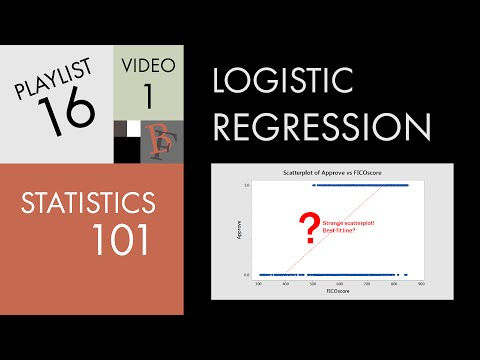Today I learned about logistic regression.

Logistic Regression is a statistical model that we can use for classification problems in machine learning.

You can easily confuse the term with linear regression.

With linear regression, you model the probability of a quantitative value, for example a price.
With logistic regression you can predict categories: yes/no, pass/fail, etc.

Logistic regression is used to describe data and to explain the relationship between one dependent binary variable and one or more nominal, ordinal, interval or ratio-level independent variables. 1

In plain English:
You have a target value that is part of a category. For example, does someone have heart disease or not? Yes/No.

You have one or more variables that you would like to put into a relationship with your target value.

For example, you know the age and maximum heart rate of the patients.

Based on that data, you can now use logistic regression to predict the probability if someone of a certain age and heart rate has heart disease or not.

Brandon Foltz has an excellent video series about the topic: